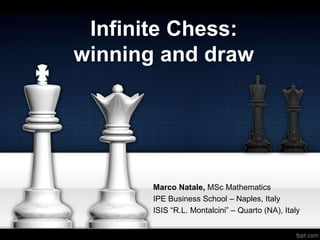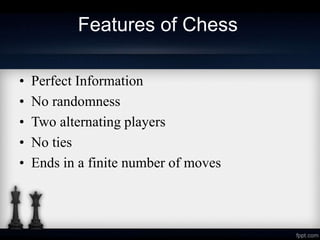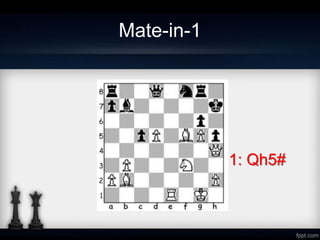Successfully reported this slideshow.

# Infinite Chess: winning and draw×

1 of 29 Ad
1 of 29 Ad

# Infinite Chess: winning and draw

A presentation about Infinite Chess and the difference between man and machines. From works by C.D.A. Evans and J.D. Hamkins. Presented during the International Interdisciplinary Seminar of London, January 2018.

A presentation about Infinite Chess and the difference between man and machines. From works by C.D.A. Evans and J.D. Hamkins. Presented during the International Interdisciplinary Seminar of London, January 2018.

## More Related Content

### Infinite Chess: winning and draw

1. 1. Infinite Chess: winning and draw Marco Natale, MSc Mathematics IPE Business School – Naples, Italy ISIS “R.L. Montalcini” – Quarto (NA), Italy
2. 2. Features of Chess • Perfect Information • No randomness • Two alternating players • No ties • Ends in a finite number of moves
3. 3. Mate-in-1 1: Qh5#
4. 4. Mate-in-2 1:Qh6+ - h6 2: Be5#
5. 5. Winning Strategy • A position in an assignment to each location on the board indicating whether or not there is a piece there and if so, which piece. A position p is said to be computable if there exists Turing machine (i.e. a deterministic procedure) able to computable it. • A strategy for one of the players from a given position p is a function that tells the player, given the prior sequence of moves from p, what he is to play next. A strategy τ is computable, if this function is a computable function in the sense of Turing computability. • If τ and σ are strategies, then τ ∗ σ indicates the play resulting from having the ﬁrst player play strategy τ and the second player play strategy σ. • The strategy τ defeats σ, if this play results in a win for the ﬁrst player. A strategy τ is a winning strategy, if it defeats all opposing strategies.
6. 6. Game Value (a.k.a. the Doomsday Clock) • A position is said to be mate-in-n for the ﬁrst player, if there is a strategy leading to checkmate in at most n moves, regardless of how the opponent plays. • In this case, we say that the Game Value is equal to n.
7. 7. Mate-in-N ??
8. 8. Features of Chess • Perfect Information • No randomness • Two alternating players • No ties • Ends in a finite number of moves Zermelo’s THeorem
9. 9. Let’s go infinite…
10. 10. Infinite Chess Features • Chess Board: infinite, edgeless (~ℤ×ℤ) – No pawn promotion, castling nor en passant • Pieces: familiar chess pieces, which can be finite or infinite in number (max. 1 king) • There is no standard starting configuration • Infinite play is a draw • No three-fold repetition rule
11. 11. Infinite Chess Mate-In-n White mates in (at most) 5 GV=5
12. 12. Infinite Chess Mate-In-n White mates in ω moves GV=…
13. 13. Releasing the Hordes The pawn columns continue inﬁnitely up and down, dividing the plane into two halves. White aims to open the portcullis — the emphasized pawn below the central bishop — thereby releasing the queen horde into the right half- plane, which can proceed to deliver checkmate.
14. 14. Releasing the Hordes White mates in ω2 moves GV=ω2
15. 15. Ordinal Numbers • In set theory, an ordinal number, is one generalization of the concept of a natural number that is used to describe a way to arrange a collection of objects in order, one after another. • Any finite collection of objects can be put in order just by the process of counting: labelling the objects with distinct whole numbers. Ordinal numbers are thus the "labels" needed to arrange collections of objects in order. • We do not need to count after infinity, just to order after infinity.
16. 16. Ordinal Numbers • In mathematics, specifically set theory, an ordinal α is said to be recursive if there is a Turing machine able to compute it (at least in principle). • It is easy to prove that ω is recursive. • The supremum of all recursive ordinal, the Church- Kleene ordinal 𝜔1 𝐶𝐾 , is non recursive. • As a consequence, an ordinal number α is recursive if and only if 𝛼 < 𝜔1 𝐶𝐾
17. 17. The “Omega One of Chess” Definition: • The ordinal ω1 𝐶ℎ is the supremum of the game values of the winning finite positions for white in infinite chess • The ordinal ω1 𝐶ℎ is the supremum of the game values of the winning positions for white in infinite chess, including positions with infinitely many pieces
18. 18. The “Omega One of Chess” • Theorem: ω1 𝐶ℎ < ω1 𝐶ℎ < ω1 𝐶𝐾 • Proof: To explain, let us review how the ordinal game values arise. – Suppose without loss of generality that white is the designated player. – Consider the game tree T of all possible ﬁnite plays, that is, ﬁnite sequences of positions, each obtained by a legal move from the previous position.
19. 19. The “Omega One of Chess” • Theorem: ω1 𝐶ℎ < ω1 𝐶ℎ < ω1 𝐶𝐾 • Proof: – Speciﬁcally, the positions with value 0 are precisely the positions in which the game has just been completed with a win for white. – If a position p is black-to-play, but all legal black plays result in a position having a previously assigned ordinal value, then the value of position p is decreed to be the supremum of these ordinals.
20. 20. The “Omega One of Chess” • Theorem: ω1 𝐶ℎ < ω1 𝐶ℎ < ω1 𝐶𝐾 • Proof: – If a position p is white-to-play, and there is a white move to a position with value β, with β minimal, then p has value β+1. – In this way, for any position p with value, we may recursively associate to p the value-reducing strategy p → v(p).
21. 21. The “Omega One of Chess” • Theorem: ω1 𝐶ℎ < ω1 𝐶ℎ < ω1 𝐶𝐾 • Conclusion: – If a position p is a winning position for white, there is a Turing machine able to compute the value-reducing strategy p → v(p), and therefore to win.
22. 22. An interesting position • Theorem: there is a position in infinite chess such that: – The position is computable, – In the category of computable play, the position is a win for white (i.e. white has a computable strategy defeating every computable strategy for black), – In the category of arbitrary play, the position is a draw.
23. 23. An interesting position • Proof: – Let T be any computable inﬁnite tree having no computable inﬁnite branch. (Such trees are known to exist by classical results). – Since the tree is inﬁnite, it is an inﬁnite ﬁnitely- branching tree, and hence it has an inﬁnite branch, even if it has no inﬁnite computable branch.
24. 24. An interesting position • The position consists of a series of channels, branching points and dead-ends, arranged in the form of a tree, whose tree structure coincides with T, • Every branch is finite, so it is computable, • Anyway, the whole tree is not computable (hint: Axiom of Choice).
25. 25. An interesting position • Because the tree T has an inﬁnite branch, then it is clear that black has a drawing strategy: simply climb the tree along an inﬁnite branch. • If black is restricted to play a computable strategy, since the tree has no computable infinite branch, black will inevitably find himself in one of the dead- end zones.
26. 26. An interesting position • If black is not restricted to play in according to a computable strategy, he can simply continue to move infinitely through the tree, therefore forcing a draw.
27. 27. Conclusion We have found a position in infinite chess such that: • if both players are required to implement computable strategies (i.e. if they are computer programs), white can force a win; • if white implements a computable strategy, while black is not required to do so, black can force a draw.
28. 28. Conclusion The draw that black can force in this position is therefore an example of something that a man could in principle do, which is not allowed to a machine.
29. 29. References • C.D.A. Evans and Joel David Hamkins. “Transﬁnite game values in inﬁnite chess”. Integers, 14: Paper No. G2, 36, 2014. • C.D.A. Evans, J. D. Hamkins, and N.L. Perlmutter, “A position in infinite chess with game value ω4,” Integers, vol. 17, p. Paper No. G4, 22, 2017.